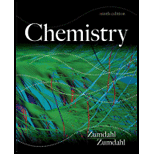# In a 1-L beaker, 203 mL of 0.307 M ammonium chromate was mixed with 137 mL of 0.269 M chromium(III) nitrite to produce ammonium nitrite and chromium(III) chromate. Write the balanced chemical equation for the reaction occurring here. If the percent yield of the reaction was 88.0%, what mass of chrornium(III) chromate was isolated?### Chemistry

9th Edition
Steven S. Zumdahl
Publisher: Cengage Learning
ISBN: 9781133611097

#### Solutions

Chapter
Section### Chemistry

9th Edition
Steven S. Zumdahl
Publisher: Cengage Learning
ISBN: 9781133611097
Chapter 4, Problem 141IP
Textbook Problem
457 views

## In a 1-L beaker, 203 mL of 0.307 M ammonium chromate was mixed with 137 mL of 0.269 M chromium(III) nitrite to produce ammonium nitrite and chromium(III) chromate. Write the balanced chemical equation for the reaction occurring here. If the percent yield of the reaction was 88.0%, what mass of chrornium(III) chromate was isolated?

Interpretation Introduction

Interpretation: The mass of chromium (III) chromate has to be isolated.

Concept Introduction:

The amount of products can be defined as the ratio of the actual yield to theoretical yield. The amount of products can be calculated by using the formula,

Amountofproduct=actualyieldtheoreticalyield

### Explanation of Solution

Explanation

Record the given data

Volume of Ammonium chromate = 203mL

Molarity of ammonium chromate = 0.307M

Volume of chromium (III) nitrate= 137mL

Molarity of chromium (III) nitrate= 0.269M

The volumes and molarities of ammonium chromate and chromium (III) nitrate are recorded as shown above.

To calculate the moles of chromium (III) chromate

Molar mass of chromium (III) chromate= 452.00g/mol

Moles of chromium (III) chromate (with ammonium chromate as reagent) =0.2030.307mol(NH4)2CrO4L×1molCr2(CrO4)33mol(NH4)2CrO4×452.00gCr2(CrO4)3molCr2(CrO4)3

= 9.39gCr2(CrO4)3

Moles of chromium (III) chromate (with chromium (III) nitrate as reagent)

=0.1370.269molCr(NO2)3L×1molCr2(CrO4)32molCr(NO2)3×452.00gCr2(CrO4)3molCr2(CrO4)3

= 8

### Still sussing out bartleby?

Check out a sample textbook solution.

See a sample solution

#### The Solution to Your Study Problems

Bartleby provides explanations to thousands of textbook problems written by our experts, many with advanced degrees!

Get Started

Find more solutions based on key concepts
Imagine that you are standing in a pharmacy comparing the Supplement Facts panels on the labels of two suppleme...

Nutrition: Concepts and Controversies - Standalone book (MindTap Course List)

Overall, the normal ratio is about 0.2 0.4 0.6 0.8

Cardiopulmonary Anatomy & Physiology

How do a solute, a solvent, and a solution differ?

Biology: The Dynamic Science (MindTap Course List)

24. Name each compound:

Chemistry In Focus

Before takeoff on an airplane, an inquisitive student on the plane dangles an iPod by its earphone wire. It han...

Physics for Scientists and Engineers, Technology Update (No access codes included)

What percentage of the world ocean is involved in wind-driven surface currents?

Oceanography: An Invitation To Marine Science, Loose-leaf Versin